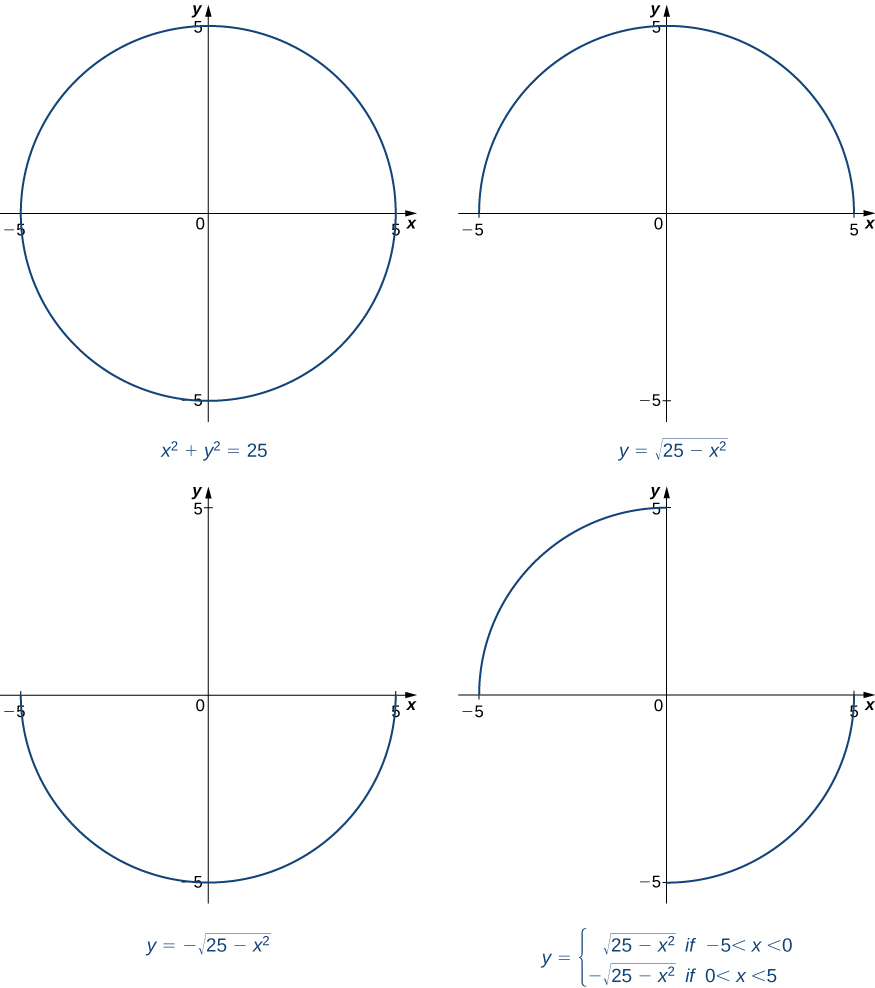# 3.8 Implicit differentiation

 Page 1 / 4
• Find the derivative of a complicated function by using implicit differentiation.
• Use implicit differentiation to determine the equation of a tangent line.

We have already studied how to find equations of tangent lines to functions and the rate of change of a function at a specific point. In all these cases we had the explicit equation for the function and differentiated these functions explicitly. Suppose instead that we want to determine the equation of a tangent line to an arbitrary curve or the rate of change of an arbitrary curve at a point. In this section, we solve these problems by finding the derivatives of functions that define $y$ implicitly in terms of $x.$

## Implicit differentiation

In most discussions of math, if the dependent variable $y$ is a function of the independent variable $x,$ we express y in terms of $x.$ If this is the case, we say that $y$ is an explicit function of $x.$ For example, when we write the equation $y={x}^{2}+1,$ we are defining y explicitly in terms of $x.$ On the other hand, if the relationship between the function $y$ and the variable $x$ is expressed by an equation where $y$ is not expressed entirely in terms of $x,$ we say that the equation defines y implicitly in terms of $x.$ For example, the equation $y-{x}^{2}=1$ defines the function $y={x}^{2}+1$ implicitly.

Implicit differentiation allows us to find slopes of tangents to curves that are clearly not functions (they fail the vertical line test). We are using the idea that portions of $y$ are functions that satisfy the given equation, but that $y$ is not actually a function of $x.$

In general, an equation defines a function implicitly if the function satisfies that equation. An equation may define many different functions implicitly. For example, the functions

$y=\sqrt{25-{x}^{2}}$ and $y=\left\{\begin{array}{c}\sqrt{25-{x}^{2}}\phantom{\rule{0.2em}{0ex}}\text{if}-25\le x<0\\ \text{−}\sqrt{25-{x}^{2}}\phantom{\rule{0.2em}{0ex}}\text{if}\phantom{\rule{0.2em}{0ex}}0\le x\le 25\end{array},$ which are illustrated in [link] , are just three of the many functions defined implicitly by the equation ${x}^{2}+{y}^{2}=25.$The equation x 2 + y 2 = 25 defines many functions implicitly.

If we want to find the slope of the line tangent to the graph of ${x}^{2}+{y}^{2}=25$ at the point $\left(3,4\right),$ we could evaluate the derivative of the function $y=\sqrt{25-{x}^{2}}$ at $x=3.$ On the other hand, if we want the slope of the tangent line at the point $\left(3,-4\right),$ we could use the derivative of $y=\text{−}\sqrt{25-{x}^{2}}.$ However, it is not always easy to solve for a function defined implicitly by an equation. Fortunately, the technique of implicit differentiation    allows us to find the derivative of an implicitly defined function without ever solving for the function explicitly. The process of finding $\frac{dy}{dx}$ using implicit differentiation is described in the following problem-solving strategy.

## Problem-solving strategy: implicit differentiation

To perform implicit differentiation on an equation that defines a function $y$ implicitly in terms of a variable $x,$ use the following steps:

1. Take the derivative of both sides of the equation. Keep in mind that y is a function of x . Consequently, whereas $\frac{d}{dx}\left(\text{sin}\phantom{\rule{0.1em}{0ex}}x\right)=\text{cos}\phantom{\rule{0.1em}{0ex}}x,\frac{d}{dx}\left(\text{sin}\phantom{\rule{0.1em}{0ex}}y\right)=\text{cos}\phantom{\rule{0.1em}{0ex}}y\frac{dy}{dx}$ because we must use the chain rule to differentiate $\text{sin}\phantom{\rule{0.1em}{0ex}}y$ with respect to $x.$
2. Rewrite the equation so that all terms containing $\frac{dy}{dx}$ are on the left and all terms that do not contain $\frac{dy}{dx}$ are on the right.
3. Factor out $\frac{dy}{dx}$ on the left.
4. Solve for $\frac{dy}{dx}$ by dividing both sides of the equation by an appropriate algebraic expression.

#### Questions & Answers

Find the derivative of g(x)=−3.
Abdullah Reply
any genius online ? I need help!!
Guzorochi Reply
how can i help you?
Pina
need to learn polynomial
Zakariya
i will teach...
nandu
I'm waiting
Zakariya
plz help me in question
Abish
evaluate the following computation (x³-8/x-2)
Murtala Reply
teach me how to solve the first law of calculus.
Uncle Reply
what is differentiation
Ibrahim Reply
f(x) = x-2 g(x) = 3x + 5 fog(x)? f(x)/g(x)
Naufal Reply
fog(x)= f(g(x)) = x-2 = 3x+5-2 = 3x+3 f(x)/g(x)= x-2/3x+5
diron
pweding paturo nsa calculus?
jimmy
how to use fundamental theorem to solve exponential
JULIA Reply
find the bounded area of the parabola y^2=4x and y=16x
Omar Reply
what is absolute value means?
Geo Reply
Chicken nuggets
Hugh
🐔
MM
🐔🦃 nuggets
MM
(mathematics) For a complex number a+bi, the principal square root of the sum of the squares of its real and imaginary parts, √a2+b2 . Denoted by | |. The absolute value |x| of a real number x is √x2 , which is equal to x if x is non-negative, and −x if x is negative.
Ismael
find integration of loge x
Game Reply
find the volume of a solid about the y-axis, x=0, x=1, y=0, y=7+x^3
Godwin Reply
how does this work
Brad Reply
Can calculus give the answers as same as other methods give in basic classes while solving the numericals?
Cosmos Reply
log tan (x/4+x/2)
Rohan
please answer
Rohan
y=(x^2 + 3x).(eipix)
Claudia
is this a answer
Ismael
A Function F(X)=Sinx+cosx is odd or even?
WIZARD Reply
neither
David
Neither
Lovuyiso
f(x)=1/1+x^2 |=[-3,1]
Yuliana Reply
apa itu?
fauzi

### Read also:

#### Get Jobilize Job Search Mobile App in your pocket Now!

Source:  OpenStax, Calculus volume 1. OpenStax CNX. Feb 05, 2016 Download for free at http://cnx.org/content/col11964/1.2
Google Play and the Google Play logo are trademarks of Google Inc.

Notification Switch

Would you like to follow the 'Calculus volume 1' conversation and receive update notifications?By Brooke DelaneyBy Yasser IbrahimBy OpenStaxBy Jonathan LongBy Sheila LopezBy Madison ChristianBy Qqq QqqBy OpenStaxBy Dionne MahaffeyBy Mike Wolf